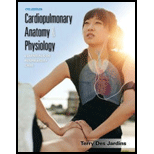# According to Laplace’s law, if a bubble with a radius of 4 cm and a distending pressure of 10 cm H 2 O is reduced to a radius of 2 cm, the new distending pressure of the bubble will be A. 5 cm H 2 O. B. 10 cm H 2 O. C. 15 cm H 2 O. D. 20 cm H 2 O.### Cardiopulmonary Anatomy & Physiolo...

7th Edition
Des Jardins + 1 other
Publisher: Cengage Learning,
ISBN: 9781337794909### Cardiopulmonary Anatomy & Physiolo...

7th Edition
Des Jardins + 1 other
Publisher: Cengage Learning,
ISBN: 9781337794909

#### Solutions

Chapter
Section
Chapter 2, Problem 12RQ
Textbook Problem

## According to Laplace’s law, if a bubble with a radius of 4 cm and a distending pressure of 10 cm H2O is reduced to a radius of 2 cm, the new distending pressure of the bubble will beA. 5 cm H2O.B. 10 cm H2O.C. 15 cm H2O.D. 20 cm H2O.

Expert Solution
Summary Introduction

Introduction:

The cohesive force that occurs at the liquid-gas interface through which the liquid molecules are strongly attracted to the molecules in the bulk of the liquid is termed as surface tension. Laplace’s law describes that the distending pressure of a liquid sphere is directly proportional to the surface tension of the liquid and inversely proportional to the radius of the sphere. This law is also applied to the alveoli of lungs because the alveoli are also in contact with each other and there also exists a surface area, which is the intra-alveolar surface area. The equation of Laplace’s law is:

Pressure, P=2STr

Where ST is the surface tension measured in dynes per centimeters and r is the radius of the liquid sphere in centimeters.

### Explanation of Solution

Justification/ Explanation for the correct answer:

Option (d) states that the new distending pressure of the bubble would be 20 cm H2O when the radius is changed from 4 cm to 2 cm, which is the correct option. The distending pressure is determined by the Laplace’s law, according to which the pressure is inversely proportional to the radius of the bubble.

P (old pressure)=2STr (old radius)=2ST4 cm

P' (new pressure)=2STr'(new radius)P'=2STr' =2ST2cm =4×2ST4×2cm

=42(2ST4cm

### Want to see the full answer?

Check out a sample textbook solution.See solution

### Want to see this answer and more?

Bartleby provides explanations to thousands of textbook problems written by our experts, many with advanced degrees!

See solution

Find more solutions based on key concepts
Show solutions
The kidneysworking units are a. photons. b. genes. c. nephrons. d. villi.

Nutrition: Concepts and Controversies - Standalone book (MindTap Course List)

What is the major difference in structure between chlorophyll and heme?

Introduction to General, Organic and Biochemistry

Why do biologists often use model organisms in their research?

Biology: The Dynamic Science (MindTap Course List)

Why are the boiling points of amines lower than those of corresponding alcohols?

Chemistry for Today: General, Organic, and Biochemistry

______ steal parental care. a. Mutualists b. Commensalists c. Brood parasites d. Predators

Biology: The Unity and Diversity of Life (MindTap Course List)

If the net work done by external forces on a particle is zero, which of the following statements about the part...

Physics for Scientists and Engineers, Technology Update (No access codes included)